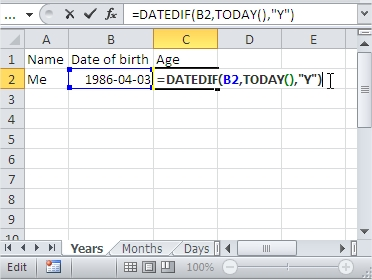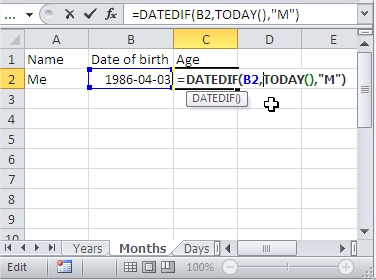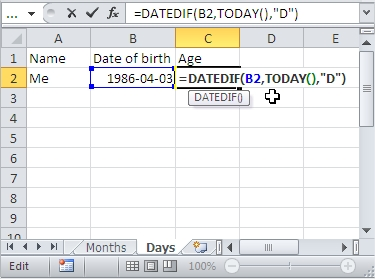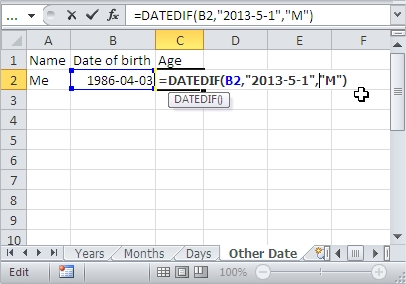#### How to Calculate Age in Excel

Calculating the date difference between two dates is a common task in Excel. This tutorial will show you how to calculate the age of a person based on their date of birth using a variety of methods.

## Datedif function

To calculate age in Excel, you can use the following formula:

=DATEDIF(birthdate, today,”unit”)

Where:

• `birthdate` is the cell containing the date of birth
• `today` is the current date, which you can get using the `TODAY()` function
• `unit` is the unit you want to use to calculate the age, which can be:
• `"y"` for years
• `"m"` for months
• `"d"` for days
• `"ym"` for the number of complete years and months
• `"md"` for the number of complete months and days

## Age calculator

To calculate the age in Excel follow these steps:

1. In the table, put the necessary data, such as name and date of birth.

2. Click on the cell where you want to see a person’s age in years (for example, it is C2). Then in the box, type the following formula:

=DATEDIF(B2,TODAY(),”Y”)

B2 is the address of a cell containing a date of birth. Press the Enter key. Age will be calculated as indicated by our cells.In the same way, you can show in Excel your age in months.

To do this, repeat steps 1 and 2, except that in the formula, instead of Y put M. The formula is the following entry:

=DATEDIF(B2,TODAY(),”M”)To calculate in Excel the number of days that have passed since a certain date, such as the date of birth, also repeat steps 1 and 2. This time the formula looks like this:

=DATEDIF(B2,TODAY(),”D”)Of course, instead of always serving the B2 cell address of the date on which Excel is to start counting. An example formula is:

=DATEDIF(B2,”2013-5-1″,”M”)## Alternative method

Another method to calculate age in Excel is to use the following formula:

=YEAR(today) – YEAR(birthdate) – IF(MONTH(today) < MONTH(birthdate) OR (MONTH(today) = MONTH(birthdate) AND DAY(today) < DAY(birthdate)), 1, 0)

Where:

• birthdate is the cell containing the date of birth
• today is the current date, which you can get using the TODAY() function

For example, to calculate someone’s age in years, you would use the following formula:

=YEAR(TODAY()) – YEAR(A2) – IF(MONTH(TODAY()) < MONTH(A2) OR (MONTH(TODAY()) = MONTH(A2) AND DAY(TODAY()) < DAY(A2)), 1, 0)

Where `A2` is the cell containing the date of birth. This formula returns the number of complete years between the birthdate and today’s date.

## INT formula

A third method to calculate age in Excel is to use the following formula:

=INT((today – birthdate) / 365.25)

Where:

• birthdate is the cell containing the date of birth
• today is the current date, which you can get using the TODAY() function

For example, to calculate someone’s age in years, you would use the following formula:

=INT((TODAY() – A2) / 365.25)

Where A2 is the cell containing the date of birth. This formula returns the number of complete years between the birthdate and today’s date. Note that 365.25 is used to account for the leap year.

## YEARFRAC formula

A fourth method to calculate age in Excel is to use the YEARFRAC function along with the INT function, as follows:

=INT(YEARFRAC(birthdate, today, “basis”))

Where:

• birthdate is the cell containing the date of birth
• today is the current date, which you can get using the TODAY() function
• “basis” is the type of year calculation you want to use, with 0 being the default and equivalent to “Actual/Actual”

For example, to calculate someone’s age in years, you would use the following formula:

=INT(YEARFRAC(A2, TODAY(), 0))

Where A2 is the cell containing the date of birth. This formula returns the number of complete years between the birthdate and today’s date.

## TEXT function

A fifth method to calculate age in Excel is to use the TEXT function along with the DATEDIF function, as follows:

=TEXT(DATEDIF(birthdate, today, “unit”), “#”)

Where:

• birthdate is the cell containing the date of birth
• today is the current date, which you can get using the TODAY() function
• “unit” is the unit you want to use to calculate the age, which can be:
• “y” for years
• “m” for months
• “d” for days
• “ym” for the number of complete years and months
• “md” for the number of complete months and days
• “#” is the format code to display the result as a number

For example, to calculate someone’s age in years, you would use the following formula:

=TEXT(DATEDIF(A2, TODAY(), “y”), “#”)

Where A2 is the cell containing the date of birth. This formula returns the number of complete years between the birthdate and today’s date.

## INT and MOD formula

A sixth method to calculate age in Excel is to use the `INT` function along with the `MOD` function, as follows:

=INT((today – birthdate) / 365.25) & ” years ” & MOD((today – birthdate), 365.25) & ” days”

Where:

• `birthdate` is the cell containing the date of birth
• `today` is the current date, which you can get using the `TODAY()` function

For example, to calculate someone’s age in years and days, you would use the following formula:

=INT((TODAY() – A2) / 365.25) & ” years ” & MOD((TODAY() – A2), 365.25) & ” days”

Where `A2` is the cell containing the date of birth. This formula returns the number of complete years and days between the birthdate and today’s date. Note that `365.25` is used to account for the leap year.